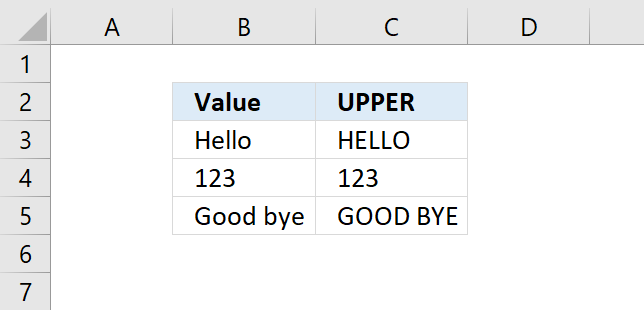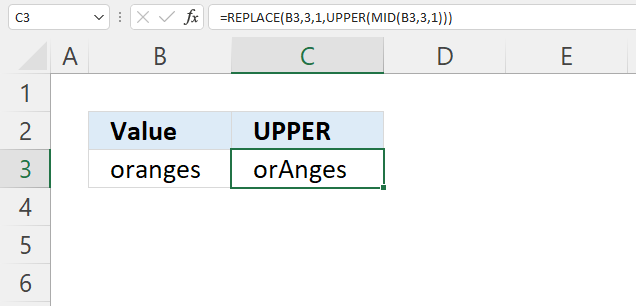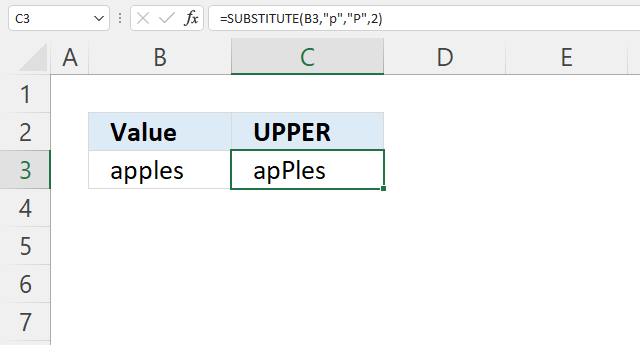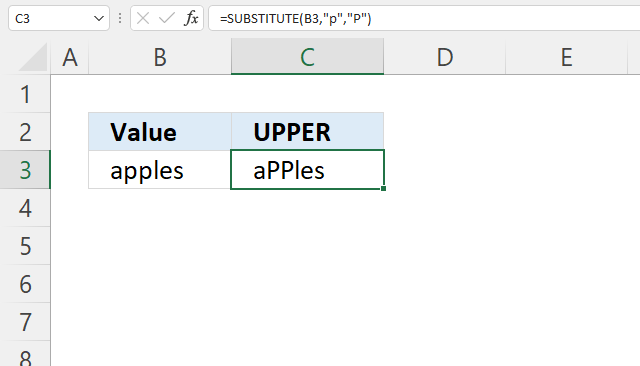Author: Oscar Cronquist Article last updated on February 24, 2022Converts a value to upper case letters.

Formula in cell C3:

=UPPER(B3)

UPPER(text)

## 2. UPPER Function Arguments

 text Value to convert. Required.

## 3. UPPER Function not working

• Check the number of arguments you use, the UPPER function accepts only one argument.

## 4. How to change lower letter to upper letter for the position of a given characterThe image above demonstrates a formula that replaces the third letter with an upper case letter.

Formula in cell C3:

=REPLACE(B3, 3, 1, UPPER(MID(B3, 3, 1)))

### 4.1 Explaining formula

#### Step 1 - Extract letter based on position

The MID function returns a substring from a string based on the starting position and the number of characters you want to extract.

MID(textstart_numnum_chars)

MID(B3, 3, 1)

becomes

MID("oranges", 3, 1)

and returns "a".

#### Step 2 - Convert to upper letter

The UPPER function converts a string to capital letters.

UPPER(MID(B3, 3, 1))

becomes

UPPER("a")

and returns "A".

#### Step 3 - Replace character based on position to an upper letter

The REPLACE function substitutes a string based on character position and length.

REPLACE(old_text, start_num, num_chars, new_text)

REPLACE(B3, 3, 1, UPPER(MID(B3, 3, 1)))

becomes

REPLACE(B3, 3, 1, "A")

becomes

REPLACE("oranges", 3, 1, "A")

and returns "orAnges".

## 5. How to change a given lower letter to an upper letterThe formula in cell C3 demonstrated in the image above converts the second lower case "p" from the right with an upper case "P".

Formula in cell C3:

=SUBSTITUTE(B3, "p", "P", 2)

### 5.1 Explaining formula

The SUBSTITUTE function substitutes a string with another string.

SUBSTITUTE(textold_textnew_text, [instance_num])

SUBSTITUTE(B3, "p", "P", 2)

The first argument is a cell reference to cell B3, the second argument is the string to be replaced.

The third argument is what will be there after the substitution and the fourth argument is a number representing the instance.

## 6. How to change all lower letters to upper letters for a given characterThe picture above shows a formula in cell C3 that converts all lower case "p" to an upper case "P" in cell B3.

Formula in cell C3:

=SUBSTITUTE(B3, "p", "P")

The SUBSTITUTE function substitutes a string with another string.

SUBSTITUTE(textold_textnew_text, [instance_num])

If the instance_num argument is omitted all instances are replaced.